## Summary

One of my favourite postprocessing effects are outlines. Doing outlines via postprocessing has many advantages. It’s better at detecting edges than the alternative (inverted hull outlines) and you don’t have to change all of your materials to give them the outline effect.

To understand how to create outlines via postprocessing it’s best to have understood how to get access to the depth and normals of the scene first.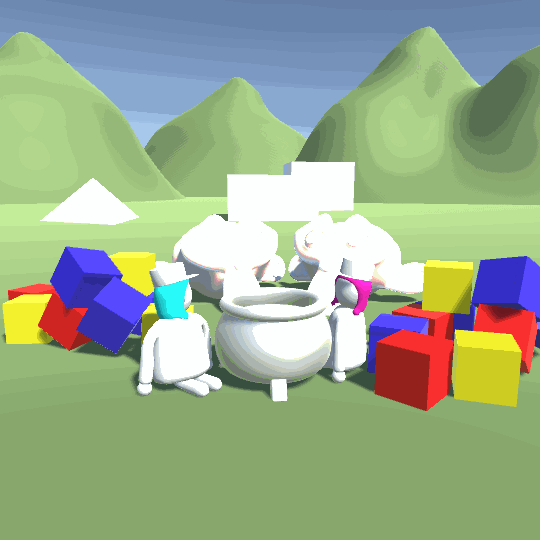## Depth Outlines

The first changes we make is to remove properties and variables which were specific to the “color on top” shader. So the cutoff value and the color. We also remove the view to world matrix, because we our outlines don‘t have a specific rotation in the world so we can ignore it. Then we remove all of the code after the part where we calculate the depth and normals.

``````//show values to edit in inspector
Properties{
[HideInInspector]_MainTex ("Texture", 2D) = "white" {}
}
``````
``````//the fragment shader
fixed4 frag(v2f i) : SV_TARGET{

//decode depthnormal
float3 normal;
float depth;
DecodeDepthNormal(depthnormal, depth, normal);

//get depth as distance from camera in units
depth = depth * _ProjectionParams.z;

}
``````

Then we remove the part where we write the camera matrix to the shader from our C# script.

``````//method which is automatically called by unity after the camera is done rendering
private void OnRenderImage(RenderTexture source, RenderTexture destination){
//draws the pixels from the source texture to the destination texture
Graphics.Blit(source, destination, postprocessMaterial);
}
``````

The way we’re going to calculate the outlines is that we’re going to read from several pixels around the pixel we’re rendering and calculate the difference in depth and normals to the center pixel. The more different they are, the stronger the outline is.

To calculate the position of the neighboring pixels we need to know how big one pixel is. Luckily we can simply add a variable with a specific name and unity tells us the size. Because technically we’re working with texture pixels, it’s called the texelsize.

We can simply create a variable called texturename_TexelSize for any texture and get the size.

``````//the depth normals texture
//texelsize of the depthnormals texture
``````

Then we copy the code for accessing the depth and normals, but change the names and we access the texture slightly to the right.

``````//read neighbor pixel
float3 neighborNormal;
float neighborDepth;
DecodeDepthNormal(neighborDepthnormal, neighborDepth, neighborNormal);
neighborDepth = neighborDepth * _ProjectionParams.z;
``````

Now that we have two samples we can calculate the difference and draw it to the screen.

``````float difference = depth - neightborDepth;
return difference;
``````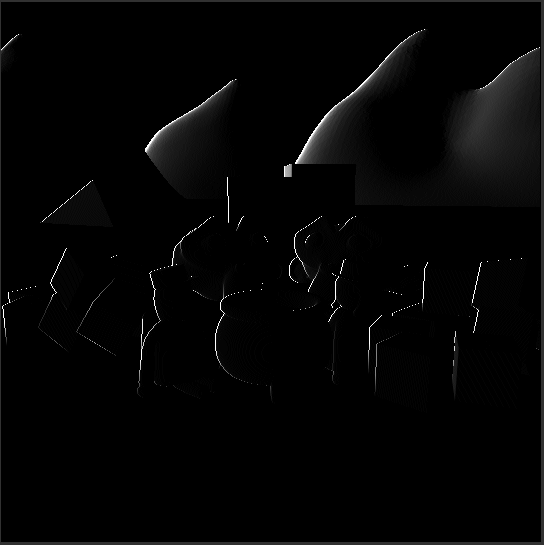With this we can already see the outlines on the left side of the objects. Before we proceed with the next sample, I’d like to put the code for reading the sample and comparing it to the center values into a separate function so we don’t have to write it 4 times. This function needs the depth of the center pixel, the uv coordinates of the center pixel and the offset as arguments. We will define the offset in pixels because that’s the easiest for us to read.

So we simply copy the code from our fragment function to the new method and replace the depth and uv names with the names of the fitting arguments. To use the offset, we multiply it with the x and y coordinates of the texel size and then add the result to the uv coordinates just like previously.

After we set up the new method we call it in the fragment method and draw the result to the screen.

``````void Compare(float baseDepth, float2 uv, float2 offset){
float3 neighborNormal;
float neighborDepth;
DecodeDepthNormal(neighborDepthnormal, neighborDepth, neighborNormal);
neighborDepth = neighborDepth * _ProjectionParams.z;

return baseDepth - neighborDepth;
}
``````
``````    float depthDifference = Compare(depth, i.uv, float2(1, 0));

return depthDifference;
}
``````

The result should look exactly like previously, but now it’s way easier to expand the shader to read samples in multiple directions. So we sample the pixels up, right and down too and add the results of all samples together.

``````//the fragment shader
fixed4 frag(v2f i) : SV_TARGET{

//decode depthnormal
float3 normal;
float depth;
DecodeDepthNormal(depthnormal, depth, normal);

//get depth as distance from camera in units
depth = depth * _ProjectionParams.z;

float depthDifference = Compare(depth, i.uv, float2(1, 0));
depthDifference = depthDifference + Compare(depth, i.uv, float2(0, 1));
depthDifference = depthDifference + Compare(depth, i.uv, float2(0, -1));
depthDifference = depthDifference + Compare(depth, i.uv, float2(-1, 0));

return depthDifference;
}
``````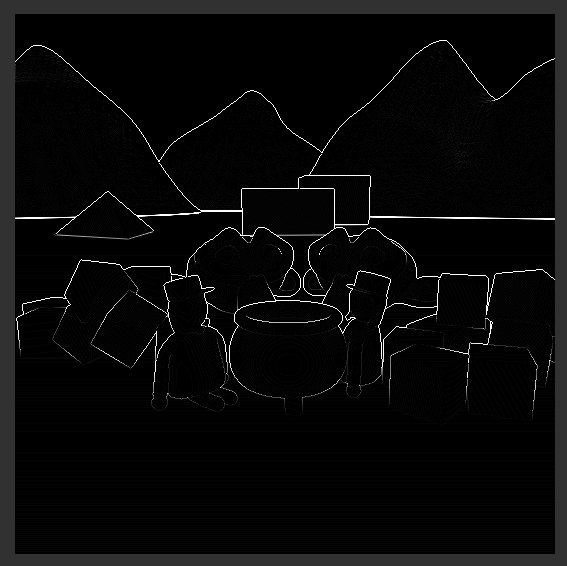## Normal Outlines

Using the depth already gives us pretty good outlines, but we can go further by also using the normals provided to us. We will also sample the normals in our compare function, but function can only return one value in hlsl so we can’t use the return value here. Instead of using the return value, we can add two new arguments with the inout keyword. With this keyword the value we pass into the function can be written to and the changes apply to the version of the variable pass in, not only the version in the function. Another thing we need to generate outlines from the normal is the outline of the center pixel, so we add that too to the list of our arguments.

``````void Compare(inout float depthOutline, inout float normalOutline,
float baseDepth, float3 baseNormal, float2 uv, float2 offset){
``````

Because we now have complete control over the outline variable we can now also do the adding to the existing outline in the method. After we changed that we go back to the fragment method, create a new variable for the difference of the normals and change the way we call the compare method to fit our new arguments.

``````void Compare(inout float depthOutline, inout float normalOutline,
float baseDepth, float3 baseNormal, float2 uv, float2 offset){
float3 neighborNormal;
float neighborDepth;
DecodeDepthNormal(neighborDepthnormal, neighborDepth, neighborNormal);
neighborDepth = neighborDepth * _ProjectionParams.z;

float depthDifference = baseDepth - neighborDepth;
depthOutline = depthOutline + depthDifference;
}
``````
``````float depthDifference = 0;
float normalDifference = 0;

Compare(depthDifference, normalDifference, depth, normal, i.uv, float2(1, 0));
Compare(depthDifference, normalDifference, depth, normal, i.uv, float2(0, 1));
Compare(depthDifference, normalDifference, depth, normal, i.uv, float2(0, -1));
Compare(depthDifference, normalDifference, depth, normal, i.uv, float2(-1, 0));

return depthDifference;
``````

This again shouldn’t change the output of the method, but the new architecture allows us to also change the difference of the normal too. A easy and fast way to compare two normalised vectors is to take the dot product. The problem about the dot product is that when the vectors point in the same direction, the dot product is 1 and when the vectors move away from each other the dot product becomes lower, the opposite of what we want. The way to fixing that is to subtract the dot product from 1. Then, when the result of the dot product is 1 the overall result is 0 and when the result of the dot product becomes lower, the overall result increases. After we calculate the normal difference, we add it to the overall difference and we change the output to show the normal difference for now.

``````float3 normalDifference = baseNormal - neighborNormal;
normalDifference = normalDifference.r + normalDifference.g + normalDifference.b;
normalOutline = normalOutline + normalDifference;
``````
``````return normalDifference;
``````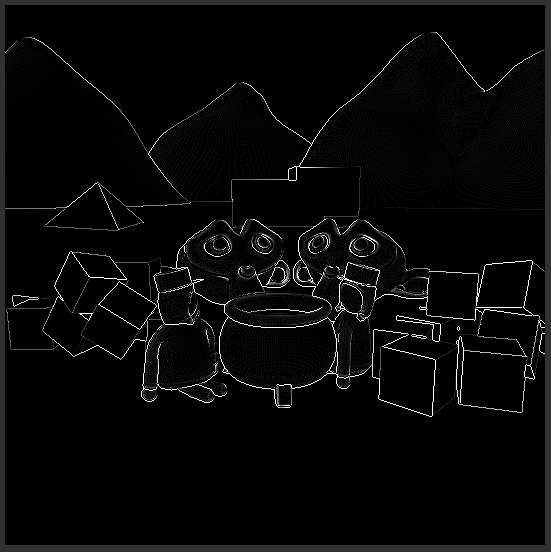With those changes we can see outlines, but they’re different outlines than before because they’re generated from the normals instead of the depth. We can then combine the two outlines to generatecombined outline.

``````return depthDifference + normalDifference;
``````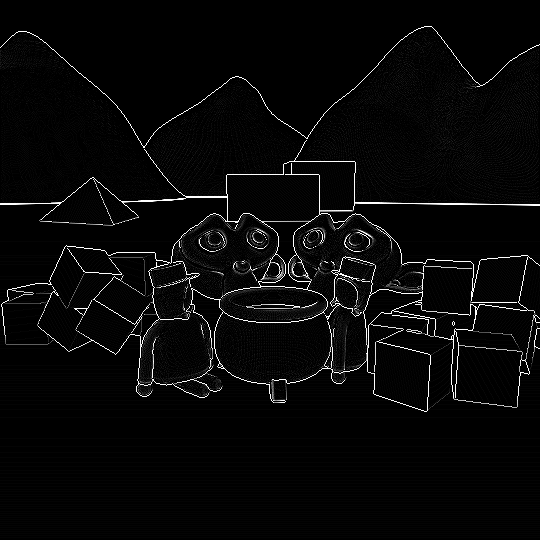## Customizable Outlines

The next step is to make the outlines more customisable. To archieve that we add two variables for each depth and normal outlines. A multiplier to make the outlines appear stronger or weaker and a bias that can make the greyish parts of the outlines we might not want vanish.

``````//show values to edit in inspector
Properties{
[HideInInspector]_MainTex ("Texture", 2D) = "white" {}
_NormalMult ("Normal Outline Multiplier", Range(0,4)) = 1
_NormalBias ("Normal Outline Bias", Range(1,4)) = 1
_DepthMult ("Depth Outline Multiplier", Range(0,4)) = 1
_DepthBias ("Depth Outline Bias", Range(1,4)) = 1
}
``````
``````//variables for customising the effect
float _NormalMult;
float _NormalBias;
float _DepthMult;
float _DepthBias;
``````

To use the variables, after adding all of the sample differences, we simply multiply the difference variables with the multipliers, then we clamp them between 0 and 1 and get the difference to the power of the bias. The clamping between 0 and 1 is important because otherwise getting the exponent of a negative number can lead to invalid results. HLSL has it’s own function for clamping a variable between 0 and 1 called “saturate”.

``````depthDifference = depthDifference * _DepthMult;
depthDifference = saturate(depthDifference);
depthDifference = pow(depthDifference, _DepthBias);

normalDifference = normalDifference * _NormalMult;
normalDifference = saturate(normalDifference);
normalDifference = pow(normalDifference, _NormalBias);

return depthDifference + normalDifference;
``````

With this you can now adjust your outlines a bit in the inspector - I boosted both normal and depth outlines a bit and reduced the noise by also increasing the bias, but it’s best to play around with the settings and see what fits your scene best.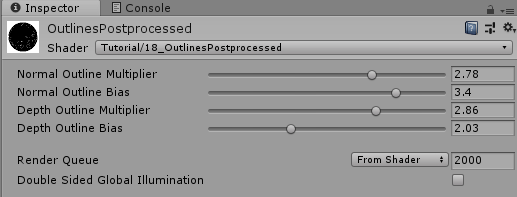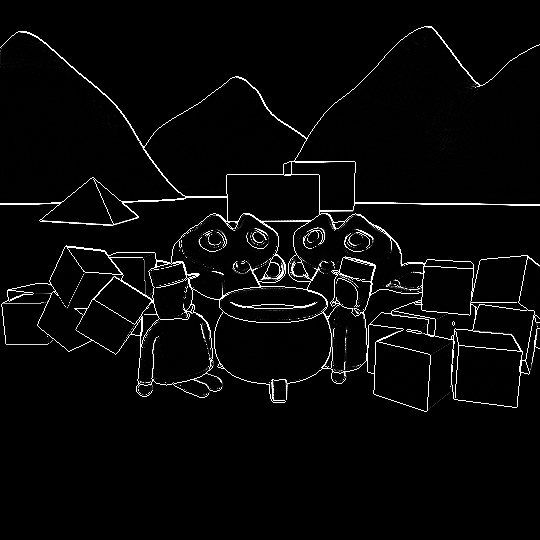Lastly we want to add our outlines to the scene, not just have them as a separate thing. For that we first declare a outline color as a property and shader variable.

``````_OutlineColor ("Outline Color", Color) = (0,0,0,1)
``````
``````float4 _OutlineColor;
``````

To apply the outlines, at the end of the fragment function, we read from the source texture and do a linear interpolation from the source color to our outline color via the combined outline, that way the pixels that were previously black are now the source color and the white ones have the outline color.

``````float outline = normalDifference + depthDifference;
float4 sourceColor = tex2D(_MainTex, i.uv);
float4 color = lerp(sourceColor, _OutlineColor, outline);
return color;
``````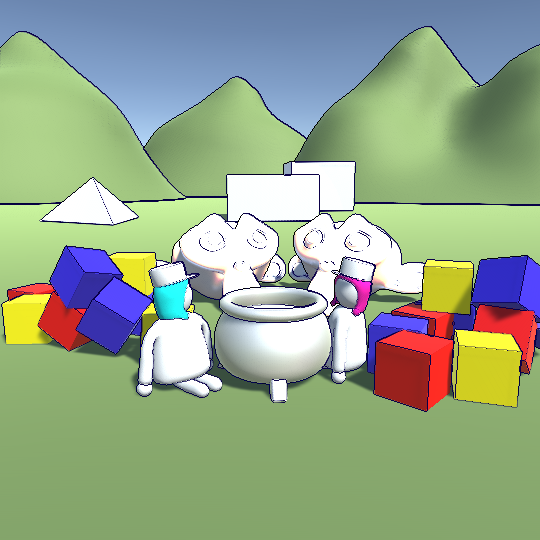The main disadvantages of postprocessed outlines are that you have to apply them to all object in the scene, The way the system decides what’s a outline and what isn’t might not fit the style your have in mind and you get aliasing (visible stairsteps) artefacts pretty quickly.

While there aren’t any easy fixes for the first two problems, you can mitigate the last one by using antialiasing in your postprocessing like FXAA or TXAA (the unity postprocessing stack provides those to you, but if you use v2 you have to redo the effect as a effect in the stack).

Another important point to keep in mind is that you have to use models that fit this way of doing outlines - if you put too much detail in your geometry the effect will paint most of your objects black, which is probably not the intended behaviour.

## Source

``````Shader "Tutorial/019_OutlinesPostprocessed"
{
//show values to edit in inspector
Properties{
[HideInInspector]_MainTex ("Texture", 2D) = "white" {}
_OutlineColor ("Outline Color", Color) = (0,0,0,1)
_NormalMult ("Normal Outline Multiplier", Range(0,4)) = 1
_NormalBias ("Normal Outline Bias", Range(1,4)) = 1
_DepthMult ("Depth Outline Multiplier", Range(0,4)) = 1
_DepthBias ("Depth Outline Bias", Range(1,4)) = 1
}

// markers that specify that we don't need culling
// or comparing/writing to the depth buffer
Cull Off
ZWrite Off
ZTest Always

Pass{
CGPROGRAM
#include "UnityCG.cginc"

#pragma vertex vert
#pragma fragment frag

//the rendered screen so far
sampler2D _MainTex;
//the depth normals texture
//texelsize of the depthnormals texture

//variables for customising the effect
float4 _OutlineColor;
float _NormalMult;
float _NormalBias;
float _DepthMult;
float _DepthBias;

//the object data that's put into the vertex shader
struct appdata{
float4 vertex : POSITION;
float2 uv : TEXCOORD0;
};

//the data that's used to generate fragments and can be read by the fragment shader
struct v2f{
float4 position : SV_POSITION;
float2 uv : TEXCOORD0;
};

v2f vert(appdata v){
v2f o;
//convert the vertex positions from object space to clip space so they can be rendered
o.position = UnityObjectToClipPos(v.vertex);
o.uv = v.uv;
return o;
}

void Compare(inout float depthOutline, inout float normalOutline,
float baseDepth, float3 baseNormal, float2 uv, float2 offset){
float3 neighborNormal;
float neighborDepth;
DecodeDepthNormal(neighborDepthnormal, neighborDepth, neighborNormal);
neighborDepth = neighborDepth * _ProjectionParams.z;

float depthDifference = baseDepth - neighborDepth;
depthOutline = depthOutline + depthDifference;

float3 normalDifference = baseNormal - neighborNormal;
normalDifference = normalDifference.r + normalDifference.g + normalDifference.b;
normalOutline = normalOutline + normalDifference;
}

fixed4 frag(v2f i) : SV_TARGET{

//decode depthnormal
float3 normal;
float depth;
DecodeDepthNormal(depthnormal, depth, normal);

//get depth as distance from camera in units
depth = depth * _ProjectionParams.z;

float depthDifference = 0;
float normalDifference = 0;

Compare(depthDifference, normalDifference, depth, normal, i.uv, float2(1, 0));
Compare(depthDifference, normalDifference, depth, normal, i.uv, float2(0, 1));
Compare(depthDifference, normalDifference, depth, normal, i.uv, float2(0, -1));
Compare(depthDifference, normalDifference, depth, normal, i.uv, float2(-1, 0));

depthDifference = depthDifference * _DepthMult;
depthDifference = saturate(depthDifference);
depthDifference = pow(depthDifference, _DepthBias);

normalDifference = normalDifference * _NormalMult;
normalDifference = saturate(normalDifference);
normalDifference = pow(normalDifference, _NormalBias);

float outline = normalDifference + depthDifference;
float4 sourceColor = tex2D(_MainTex, i.uv);
float4 color = lerp(sourceColor, _OutlineColor, outline);
return color;
}
ENDCG
}
}
}
``````
``````using UnityEngine;
using System;

//behaviour which should lie on the same gameobject as the main camera
public class OutlinesPostprocessed : MonoBehaviour {
//material that's applied when doing postprocessing
[SerializeField]
private Material postprocessMaterial;

private Camera cam;

private void Start(){
//get the camera and tell it to render a depthnormals texture
cam = GetComponent<Camera>();
cam.depthTextureMode = cam.depthTextureMode | DepthTextureMode.DepthNormals;
}

//method which is automatically called by unity after the camera is done rendering
private void OnRenderImage(RenderTexture source, RenderTexture destination){
//draws the pixels from the source texture to the destination texture
Graphics.Blit(source, destination, postprocessMaterial);
}
}
``````

I hope I was able to show you how to add nice outlines to your game and how it works.

You can also find me on twitter at @totallyRonja. If you liked my tutorial and want to support me you can do that on Patreon (patreon.com/RonjaTutorials) or Ko-Fi (ko-fi.com/RonjaTutorials).